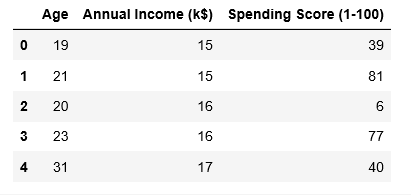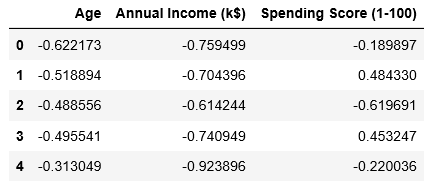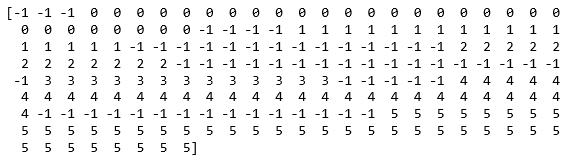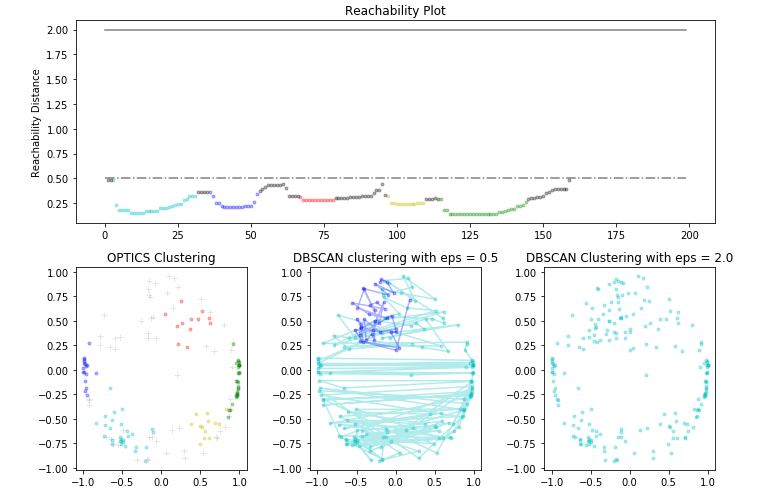Open in App
Not now

# ML | OPTICS Clustering Implementing using Sklearn

• Last Updated : 15 Feb, 2023

Prerequisites: OPTICS Clustering This article will demonstrate how to implement OPTICS Clustering technique using Sklearn in Python. The dataset used for the demonstration is the Mall Customer Segmentation Data which can be downloaded from Kaggle

Step 1: Importing the required libraries

OPTICS (Ordering Points To Identify the Clustering Structure) is a density-based clustering algorithm that is used to identify the structure of clusters in high-dimensional data. It is similar to DBSCAN, but it also produces a cluster ordering that can be used to identify the density-based clusters at multiple levels of granularity.

The implementation of OPTICS clustering using scikit-learn (sklearn) is straightforward. You can use the OPTICS class from the sklearn.cluster module. Here is an example of how to use it:

## Python

 `from` `sklearn.cluster ``import` `OPTICS``import` `numpy as np` `# Generate sample data``np.random.seed(``0``)``X ``=` `np.random.randn(``100``, ``2``)` `# Initialize the OPTICS clustering model``optics ``=` `OPTICS(min_samples``=``5``, xi``=``0.05``, min_cluster_size``=``0.05``)` `# Fit the model to the data``optics.fit(X)` `# Get the cluster labels``labels ``=` `optics.labels_`

In this example, the min_samples parameter controls the minimum number of samples required to form a dense region, the xi parameter controls the maximum distance between two samples to be considered as a neighborhood, and the min_cluster_size parameter controls the minimum size of a dense region to be considered as a cluster.

The fit method is used to fit the model to the data, and the labels_ attribute is used to get the cluster labels for each sample in the data.

Note that the implementation of OPTICS clustering in scikit-learn is based on the original paper by Ankerst, Mihael, and Markus (1999). You might consider reading this paper to learn more about the algorithm.

## Python3

 `import` `numpy as np``import` `pandas as pd``import` `matplotlib.pyplot as plt``from` `matplotlib ``import` `gridspec``from` `sklearn.cluster ``import` `OPTICS, cluster_optics_dbscan``from` `sklearn.preprocessing ``import` `normalize, StandardScaler`

## Python3

 `# Changing the working location to the location of the data``cd C:\Users\Dev\Desktop\Kaggle\Customer Segmentation` `X ``=` `pd.read_csv(``'Mall_Customers.csv'``)` `# Dropping irrelevant columns``drop_features ``=` `[``'CustomerID'``, ``'Gender'``]``X ``=` `X.drop(drop_features, axis ``=` `1``)` `# Handling the missing values if any``X.fillna(method ``=``'ffill'``, inplace ``=` `True``)` `X.head()`Step 3: Preprocessing the Data

## Python3

 `# Scaling the data to bring all the attributes to a comparable level``scaler ``=` `StandardScaler()``X_scaled ``=` `scaler.fit_transform(X)` `# Normalizing the data so that the data``# approximately follows a Gaussian distribution``X_normalized ``=` `normalize(X_scaled)` `# Converting the numpy array into a pandas DataFrame``X_normalized ``=` `pd.DataFrame(X_normalized)` `# Renaming the columns``X_normalized.columns ``=` `X.columns` `X_normalized.head()`Step 4: Building the Clustering Model

## Python3

 `# Building the OPTICS Clustering model``optics_model ``=` `OPTICS(min_samples ``=` `10``, xi ``=` `0.05``, min_cluster_size ``=` `0.05``)` `# Training the model``optics_model.fit(X_normalized)`

Step 5: Storing the results of the training

## Python3

 `# Producing the labels according to the DBSCAN technique with eps = 0.5``labels1 ``=` `cluster_optics_dbscan(reachability ``=` `optics_model.reachability_,``                                   ``core_distances ``=` `optics_model.core_distances_,``                                   ``ordering ``=` `optics_model.ordering_, eps ``=` `0.5``)` `# Producing the labels according to the DBSCAN technique with eps = 2.0``labels2 ``=` `cluster_optics_dbscan(reachability ``=` `optics_model.reachability_,``                                   ``core_distances ``=` `optics_model.core_distances_,``                                   ``ordering ``=` `optics_model.ordering_, eps ``=` `2``)` `# Creating a numpy array with numbers at equal spaces till``# the specified range``space ``=` `np.arange(``len``(X_normalized))` `# Storing the reachability distance of each point``reachability ``=` `optics_model.reachability_[optics_model.ordering_]` `# Storing the cluster labels of each point``labels ``=` `optics_model.labels_[optics_model.ordering_]` `print``(labels)`Step 6: Visualizing the results

## Python3

 `# Defining the framework of the visualization``plt.figure(figsize ``=``(``10``, ``7``))``G ``=` `gridspec.GridSpec(``2``, ``3``)``ax1 ``=` `plt.subplot(G[``0``, :])``ax2 ``=` `plt.subplot(G[``1``, ``0``])``ax3 ``=` `plt.subplot(G[``1``, ``1``])``ax4 ``=` `plt.subplot(G[``1``, ``2``])` `# Plotting the Reachability-Distance Plot``colors ``=` `[``'c.'``, ``'b.'``, ``'r.'``, ``'y.'``, ``'g.'``]``for` `Class, colour ``in` `zip``(``range``(``0``, ``5``), colors):``    ``Xk ``=` `space[labels ``=``=` `Class]``    ``Rk ``=` `reachability[labels ``=``=` `Class]``    ``ax1.plot(Xk, Rk, colour, alpha ``=` `0.3``)``ax1.plot(space[labels ``=``=` `-``1``], reachability[labels ``=``=` `-``1``], ``'k.'``, alpha ``=` `0.3``)``ax1.plot(space, np.full_like(space, ``2.``, dtype ``=` `float``), ``'k-'``, alpha ``=` `0.5``)``ax1.plot(space, np.full_like(space, ``0.5``, dtype ``=` `float``), ``'k-.'``, alpha ``=` `0.5``)``ax1.set_ylabel(``'Reachability Distance'``)``ax1.set_title(``'Reachability Plot'``)` `# Plotting the OPTICS Clustering``colors ``=` `[``'c.'``, ``'b.'``, ``'r.'``, ``'y.'``, ``'g.'``]``for` `Class, colour ``in` `zip``(``range``(``0``, ``5``), colors):``    ``Xk ``=` `X_normalized[optics_model.labels_ ``=``=` `Class]``    ``ax2.plot(Xk.iloc[:, ``0``], Xk.iloc[:, ``1``], colour, alpha ``=` `0.3``)``    ` `ax2.plot(X_normalized.iloc[optics_model.labels_ ``=``=` `-``1``, ``0``],``        ``X_normalized.iloc[optics_model.labels_ ``=``=` `-``1``, ``1``],``       ``'k+'``, alpha ``=` `0.1``)``ax2.set_title(``'OPTICS Clustering'``)` `# Plotting the DBSCAN Clustering with eps = 0.5``colors ``=` `[``'c'``, ``'b'``, ``'r'``, ``'y'``, ``'g'``, ``'greenyellow'``]``for` `Class, colour ``in` `zip``(``range``(``0``, ``6``), colors):``    ``Xk ``=` `X_normalized[labels1 ``=``=` `Class]``    ``ax3.plot(Xk.iloc[:, ``0``], Xk.iloc[:, ``1``], colour, alpha ``=` `0.3``, marker ``=``'.'``)``      ` `ax3.plot(X_normalized.iloc[labels1 ``=``=` `-``1``, ``0``],``        ``X_normalized.iloc[labels1 ``=``=` `-``1``, ``1``],``       ``'k+'``, alpha ``=` `0.1``)``ax3.set_title(``'DBSCAN clustering with eps = 0.5'``)` `# Plotting the DBSCAN Clustering with eps = 2.0``colors ``=` `[``'c.'``, ``'y.'``, ``'m.'``, ``'g.'``]``for` `Class, colour ``in` `zip``(``range``(``0``, ``4``), colors):``    ``Xk ``=` `X_normalized.iloc[labels2 ``=``=` `Class]``    ``ax4.plot(Xk.iloc[:, ``0``], Xk.iloc[:, ``1``], colour, alpha ``=` `0.3``)``        ` `ax4.plot(X_normalized.iloc[labels2 ``=``=` `-``1``, ``0``],``        ``X_normalized.iloc[labels2 ``=``=` `-``1``, ``1``],``       ``'k+'``, alpha ``=` `0.1``)``ax4.set_title(``'DBSCAN Clustering with eps = 2.0'``)`  `plt.tight_layout()``plt.show()`My Personal Notes arrow_drop_up